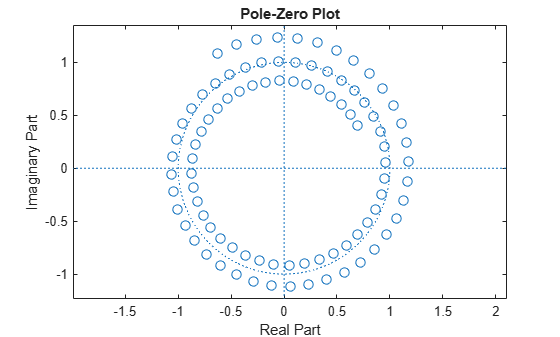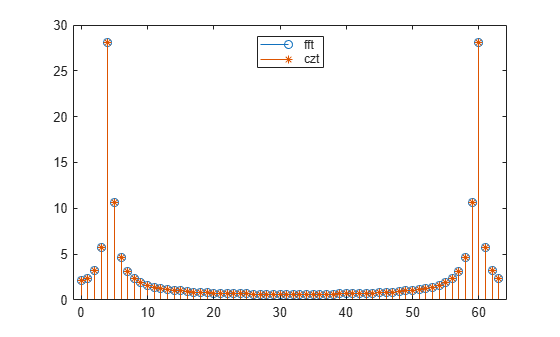Chirp Z-Transform

The chirp Z-transform (CZT) is useful in evaluating the Z-transform along contours other than the unit circle. The chirp Z-transform is also more efficient than the DFT algorithm for the computation of prime-length transforms, and it is useful in computing a subset of the DFT for a sequence. The chirp Z-transform, or CZT, computes the Z-transform along spiral contours in the z-plane for an input sequence. Unlike the DFT, the CZT is not constrained to operate along the unit circle, but can evaluate the Z-transform along contours described by ${z}_{\ell }=A{W}^{-\ell },\phantom{\rule{0.2777777777777778em}{0ex}}\ell =0,\cdots ,M-1$, where A is the complex starting point, W is a complex scalar describing the complex ratio between points on the contour, and M is the length of the transform.

One possible spiral is

a = 0.8*exp(1j*pi/6);
w = 0.995*exp(-1j*pi*.05);
m = 91;
z = a*(w.^(-(0:m-1)'));
zplane(z)czt(x,m,w,a) computes the Z-transform of x on these points.

An interesting and useful spiral set is m evenly spaced samples around the unit circle, parameterized by $A=1$ and $W=\mathrm{exp}\left(-j\pi /M\right)$. The Z-transform on this contour is simply the DFT, obtained by czt:

M = 64;
m = 0:M-1;

x = sin(2*pi*m/15);
FFT = fft(x);
CZT = czt(x,M,exp(-2j*pi/M),1);

stem(m,abs(FFT))
hold on
stem(m,abs(CZT),'*')
hold off
legend('fft','czt','Location','north')czt may be faster than the fft function for computing the DFT of sequences with certain odd lengths, particularly long prime-length sequences.